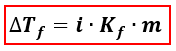# Problem: Assuming 100% dissociation, calculate the freezing point and boiling point of 2.96 m Na2SO4(aq).

🤓 Based on our data, we think this question is relevant for Professor Tisko's class at UN OMAHA.

###### FREE Expert Solution

We’re being asked to determine the freezing point and boiling point of an aqueous Na2SO4 solution. Aqueous means that Na2SO4 was dissolved in water.

When calculating the freezing point of a solution, we’re going to use the Freezing Point Depression equation. On the other hand, when calculating for the boiling point of a solution we’re going to use the Boiling Point Elevation equation.

Freezing Point Depression:∆Tf = change in freezing point = Tf pure solvent –Tf solution
Kf = freezing point depression constant
i = van' t Hoff factor of the solute = no. of ions
m = molality

Boiling Point Elevation:###### Problem Details

Assuming 100% dissociation, calculate the freezing point and boiling point of 2.96 m Na2SO4(aq).

What scientific concept do you need to know in order to solve this problem?

Our tutors have indicated that to solve this problem you will need to apply the Freezing Point Depression concept. If you need more Freezing Point Depression practice, you can also practice Freezing Point Depression practice problems.

What is the difficulty of this problem?

Our tutors rated the difficulty ofAssuming 100% dissociation, calculate the freezing point and...as high difficulty.

How long does this problem take to solve?

Our expert Chemistry tutor, Dasha took 6 minutes and 23 seconds to solve this problem. You can follow their steps in the video explanation above.

What professor is this problem relevant for?

Based on our data, we think this problem is relevant for Professor Tisko's class at UN OMAHA.